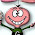Sunday, November 22, 2015

No Controversy Post on The Eight Queens Problem

This blog is going to take the place of our third blog post. The one topic that I haven’t covered yet is communicating mathematics. I doubt this will be as controversial of a piece as the previous post was since it will be more informative and less opinion based. So if one is reading this looking for something similar, I apologize.

In looking back through our course page on different thinkers around the time of the third blog post I was drawn to Thabit ibn Qurra since I knew so little about him. I wanted to delve into his mathematics for my blog. Looking at his Wikipedia page, I noticed a link to a chess solution. From that page I found a link to the eight queens puzzle. Following my interest and Wikipedia links I decided to settle on this as my topic for this blog.

The problem is as follows. Is there a way to place eight queens on a standard chessboard so that none of them could attack each other either diagonally, horizontally, or vertically? The history of this problem is part of my interest.

Max Bezzel first introduced the puzzle in 1848 in a German magazine. Over the next six years there were a variety of solutions published in various magazines. With a reposing of the question the problem shifted to a question of how many different solutions to the problem there were. Franz Nauck was the one responsible for this reposing and asserts that there were 60 solutions to the problem. This sparked a debate between two friends Gauss and Schumacher. They communicated with letters for around a month. During that time Nauck corrected himself and affirmed that there were actually 92 solutions, but offered no proof of this assertion. Gauss referred to Nauck’s correction and laid out how to go about proving it, though never actually proves it. It seems that this task wasn’t of extreme importance to Gauss since he didn’t spend the time to solve it. These letters were published about fifteen years later.

The solution that Gauss proposed was clever. I’ll try to communicate as best I can the solution that was proposed. Gauss reformulates this problem into an arithmetic one. Gauss begins by assigning a position to each permutation of the numbers one through eight. Thus 12345678 would have the first queen at a1, the second queen at b2, the third queen at c3, and so on. The permutation 45632178 would have the first queen at a4, the second queen at b5, the third queen at c6, and so on. Using this idea of position Gauss was able to work with the solutions more easily. He found that if each permutation was summed with 12345678 and separately with 87654321, and if these sums were all different in each number that this was a solution to the problem. This is difficult to understand without an example so I will share the example that Gauss sent Schumacher.

All these sums are different in this permutation of 15863724. This means that it is a solution to the eight queens problem. In the next permutation of 13425867 we can see that 10, 9, and 8 all appear twice. This means that it is not a solution to the problem.

By setting the problem up this way Gauss was able to more quickly sift through solutions. The setup itself prevents queens from lying on the same row or column. The addition of the permutation 12345678 prevents queens from lining up diagonally to the right; the addition of the permutation 87654321 prevents the queens lining up diagonally to the left.

The solution isn’t flushed out arithmetically that I could find, but was explained to have 12 fundamentally different solutions that, through rotations, are flushed into all 92 solutions. This geometric solution is nice, but I wanted the arithmetic solution.

The problem continued to evolve into boards of differing sizes and got into complications that I won’t flush out. As stated earlier, Gauss’ passion didn’t seem to be evident in this problem since he didn’t bother to create every permutation, or offer an argument for why there were only 92 solutions. I wish that his argument had been clearer and that Gauss had had more passion in the subject. This was a fun problem to post on because it combines my passion for chess and math!

1 comment:

1.I have a good chess math teacher story if you're interested.

Nice exposition. Did you try the problem before reading up on solutions? (I never have. GeoGebra if I had time....) That would be an interesting addition. An image of one of the 12 would be interesting here. (complete)

Other Cs: +

Flushed?